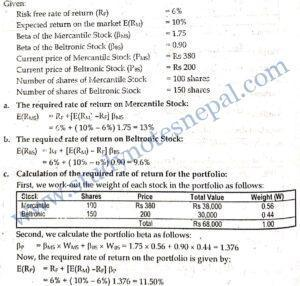###### 1. How do you distinguish a growth portfolio from an income portfolio?

A growth portfolio is one that offers a long-term capital appreciation to the investor. The example of growth portfolio is the portfolio of high growth common stock. On the other hand, the income portfolio ensures regular income such as the portfolio of fixed income securities.

###### 2. Explain the concept of efficient portfolio.

A portfolio refers to the combination of wealth into more than one asset. The objective of forming portfolio is to form an efficient portfolio of investment. A portfolio is said to be the efficient if it offers higher returns for a given level of risk or it has lower risk for a given level of return.

###### 3. Explain the concept of diversification with illustration.

Diversification refers to the combination of the investment into different assets. According to the concept of diversification, investor can reduce the investment risk of portfolio by combining the wealth into different assets that are less than perfectly positively correlated. This concept of diversification is popularly known as Markowitz diversification, which advocates that combining assets with lower correlation in a portfolio can better diversify the risk. For example, if we hold only one asset we will loose every thing if asset performs poorly. However, if we diversify the investment into more assets, it is less likely that all assets perform poorly at the same time. Therefore, by diversifying investment into more assets, portfolio risk can be minimized.

###### 4. Average return on Stock A is 12 percent while that on Stock B is 18 percent. What is the average return on your portfolio comprising equal investment in Stock A and Stock B?

SOLUTION

Given:

Average return on Stock A, E(RA) = 12%

Average return on Stock B, E(RB) = 18%

Portfolio weight of Stock A (WA) = 0.5

Portfolio weight of Stock B (WB) = 0.5

The average rate of return on the portfolio is given by:

E(RP) = WA X E(RA) + WB X E(RB)

= 0.5 x 12% +0.5 × 18%

= 6% + 9%

= 15%

###### 5. Stock X and Y both have 15 percent expected return. However, beta of Stock X is 1.2 and that of Stock Y is 1.5. Based on these information, which stock would you prefer? Why?

SOLUTION

Both Stocks have 15 percent expected return. However, the level of systematic risk measured by beta is lower for Stock X. Therefore we prefer to invest in Stock X as it has lower risk for a given level of return than Stock Y.

###### 1. Explain the contribution of Markowitz in the portfolio theory.

The main objective of Markowitz model of modern portfolio theory is to form an optimal portfolio. An optimal portfolio refers to the portfolio that offers maximum satisfaction to an investor given her/his risk tolerance. The selection of an optimal portfolio is based on investor’s utility function that represents the level of satisfaction. The optimal portfolio for an investor is based on her/his risk-return preference function derived from the concept of indifference curve. An indifference curve is the locus of points each representing a different combination of risk and return, which yield the same utility or level of satisfaction. An individual have more than one indifference curve. Each upper indifference curve represents higher level of satisfaction because for a given level of risk each risk-return combination in upper indifference curve offers higher level of returns than the lower indifference curve. The theory of indifference curve literally provides a theory of choice. Assuming all investor are risk averse, they choose higher return to accept greater risk.

Selection of optimal portfolio is also based on the concept of portfolio opportunity set and the efficient set. The portfolio opportunity set represents the set of all those possible portfolios each having different combination of risk and return that can be formed with a given amount of wealth. Figure 1 shows the set of portfolios with several risk-return combinations that can be formed by a given level of investment. The shaded area in the Figure 1 is the opportunity set of

portfolios. An investor can invest in any of the portfolios within this attainable set. The efficient set of portfolio is comprised of efficient frontier. It represents the set of portfolios in attainable set which offer maximum return for each given class of risk. From among the portfolios in opportunity set of Figure 1, the efficient frontier represents all the portfolios that place along the bold line ABD. All the portfolios in efficient frontier dominate all other portfolios in the attainable set in terms of risk or return. For example, consider portfolio S in the attainable set. It offers same level of return as to the portfolio A in the efficient frontier. However, portfolio A is less risky than S. Therefore portfolio A dominates portfolio S in terms of risk. Again compare portfolio S against the portfolio B in the efficient frontier. Both of these portfolios have same level of risk. But portfolio B dominates the portfolio S in terms of return. Thus, portfolio A is preferable than portfolio S when compared the risk. Similarly, portfolio B is preferable than portfolio S when compared the return. A risk-averse investor always chooses the portfolios that place along efficient frontier.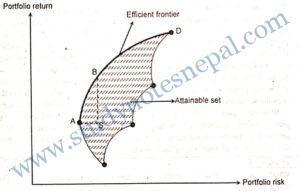Figure 1: The portfolio opportunity set

Based on the indifference curve and efficient frontier, now we can easily determine the portfolio that suits the best for an individual investor. For this purpose, we combine the investor’s utility preference curve to the efficient frontier as shown in Figure 2.

Figure 2 illustrates the optimal portfolio choice for an investor with given attitude toward risk as represented by her/his indifference curve. All portfolios on the efficient frontier necessarily are not equally likely for an investor. The portfolio on the efficient frontier that maximizes investor’s utility represents the best choice. Such portfolio is called the optimal portfolio for an investor. The optimal portfolio is determined at the point where an investor’s indifference curve is tangent to the efficient frontier. In Figure 2, this point is labeled as ‘O’. For the investor, portfolio O represents the utility maximizing portfolio among all feasible portfolios for her/him. To make it clear why the portfolio O is the optimal portfolio, let us consider portfolio B and C on the efficient frontier. Both of these portfolios are efficient portfolios and provide same level of utility because they place on the same indifference curve IC₁.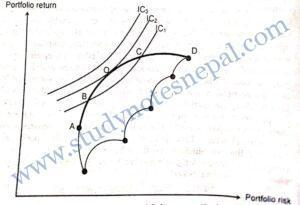Figure 2: The optimal portfolio

An investor, however, does not stop at IC, because s/he has the opportunity to form the portfolio along the upper indifference curve IC₂. Any portfolio along IC₂ further maximizes the utility to investor. There is only one feasible portfolio at this indifference curve, which is given by tangency point (portfolio O) between IC₂ and the efficient frontier. Now again consider the possibility of any portfolio along upper indifference curve IC3, which further maximizes the utility to investor than any portfolio along IC₂. In this example, portfolio along IC3 is not possible for this particular investor. IC3 completely lies beyond the feasible set of the portfolios meaning that no portfolio can be formed along this indifference curve with given level of investment opportunities. Thus, portfolio O is the utility maximizing optimal portfolio for this investor. Hence, investor needs the information about utility function and efficient frontier of the portfolio to identify the optimal portfolio.

###### 2. What is portfolio diversification? How does it help an investor?

Portfolio diversification is the process of combining different assets to reduce investment risk of portfolio. According to portfolio theory, an investment in single asset may be very risky, but if we diversify our investment into different assets by forming a portfolio, the portfolio may not be as risky as the single asset. It happens because when the return from a single asset turns out to be lower, we tend to loose from our investment in single asset. Instead, if we hold a portfolio of assets, the returns from all assets in the portfolio should be lower to experience a loss from the portfolio of assets. Therefore, by adding more and more securities in a portfolio, we can reduce investment risk to a greater extent. Thus, portfolio diversification is concerned with minimizing portfolio risk by combining investment into more than one asset.

The concept of diversification was mainly popularized by Harry Markowitz. It is known as Markowitz diversification. According to this concept, an investor can achieve the benefit of risk reduction by combining assets having lower correlation in a portfolio. More specifically, lower the correlation between assets, higher will be the benefits of risk reduction from portfolio. Therefore, the concept and techniques of diversification helps investor to reduce investment risk of a portfolio of assets. To understand how diversification helps, we need to understand the role of correlation between the assets. If correlation between the assets in a portfolio is perfectly positive, the returns from two assets move exactly into the same direction. Forming portfolio of such assets does not produce the benefits of risk reduction. However, if returns from two assets move into opposite direction, it implies that when return from one asset increases the return from other asset decreases. Due to the combine effect of returns moving into opposite direction, the volatility of overall portfolio returns will be lower. Thus, the concept of portfolio diversification suggests the investor to choose assets in a portfolio that are negatively correlated.

###### 3. Explain the risk-averse investor’s attitude towards risk.

A risk-averse investor prefers higher return and lower risk. In other words, a risk-averse investor dislikes risk. Given the choice of two investment alternatives having same level of return but different risk, the risk-averse investor prefers one having lower risk. Putting it in other way, given the choice of two investment alternatives with different level of return but equal risk, the risk-averse investor prefers the one with higher returns. There may be other investors who are risk-seeker or risk indifferent. However, the logics and observations show that almost all investors are risk-averse. Risk aversion is a common behavior for all investors. Risk aversion holds true on the part of all investors simply because of diminishing marginal utility of wealth. According to this theory, the additional utility that an investor gets from an increase in wealth is lower than the reduction in utility that the investor experiences from equal amount of decrease in wealth. Thus, primarily due to the diminishing marginal utility of wealth, investors are risk averse. However, the degree of risk aversion varies from investor to investor. Some investors may be highly risk-averse while others may be less risk- averse. A highly risk averse investor prefers lower risk-lower return combination while a less risk-averse investor prefers higher-risk higher return combination. For example, highly risk averse investors tend to choose bond investment while less risk-averse investors tend to choose common stock investment.

###### 4. How is indifference curve useful to make investment decision?

An indifference curve is the locus of points each representing a different combination of risk and return, which yield the same utility or level satisfaction. Let us consider the Figure below. IC₁ represents a locus of points representing different risk-return combination. Point a and b on IC₁ have different risk-return combinations but they lie on the same indifference curve. So, they yield same utility for the particular investor. An individual has more than one indifference curve. Each upper indifference curve represents higher level of satisfaction because, for a given level of risk, each risk-return combination in upper indifference curve offers higher level of returns than the lower indifference curve. For example consider IC₂ in the figure. It lies above IC₁. Each point on IC₂ offers higher utility to the investor. If we compare point c on IC₂ and point a on IC₁, both have same level of risk. But the point c the on the IC₂ offers higher returns. Thus any risk-return combination along IC₂ offers higher utility to investor than any point along IC₁.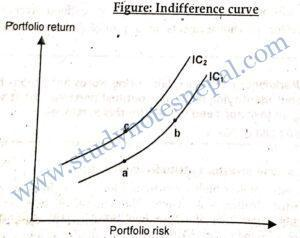The theory of indifference curve literally provides a theory of choice. A risk averse investor always prefers higher returns to accept grater risk. For a given level of risk, if any investment or portfolio offers higher return, it will maximize the investor’s utility. Thus, the theory of indifference curve is important in selecting the utility maximizing investment alternative for investors.

###### 5. What is investment risk? How is risk diversified?

Risk refers to the chance of occurring unfavourable outcomes. Investment risk refers to the chance that an investment may not produce desirable outcomes. Literally, the investment risk is defined as variability in returns. In other words, risk is the chance that an investment will fail to generate the returns as expected. Investment risk is undesirable for all investors so they try to avoid it. Risk can be diversified by forming an efficient portfolio of investment. Portfolio risk diversification is the process of combining different assets to reduce investment risk of portfolio. According to portfolio theory, an investment in single asset may be very risky, but if we diversify our investment into different assets by forming a portfolio, the portfolio may not be as risky as the single asset. It happens because when the return from a single asset turns out to be lower, we tend to loose from our investment in single asset. Instead, if we hold a portfolio of assets, the returns from all assets in the portfolio should be lower to experience a loss from the portfolio of assets. Therefore, by adding more securities in a portfolio, we can reduce investment risk to a greater extent. Thus, portfolio diversification is concerned with minimizing portfolio risk by combining investment into more than one asset.

The concept of diversification was mainly popularized by Harry Markowitz. It is known as Markowitz diversification. According to this concept, an investor can achieve the benefit of risk reduction by combining assets having lower correlation in a portfolio. More specifically, lower the correlation between assets, higher will be the benefits of risk reduction from portfolio. Therefore, the concept and techniques of diversification helps investor to reduce investment risk of a portfolio of assets. To understand how diversification helps, we need to understand the role of correlation between the assets. If correlation between the assets in a portfolio is perfectly positive, the returns from two assets move exactly into the same direction. Forming portfolio of such assets does not produce the benefits of risk reduction. However, if returns from two assets move into opposite direction, it implies that when return from one asset increases the return from other asset decreases. Due to the combine effect of returns moving into opposite direction, the volatility of overall portfolio returns will be lower. Thus, the concept of portfolio diversification suggests the investor to choose assets in a portfolio that are negatively correlated.

###### 6. Given that the expected return on the market portfolio is 10 percent, the risk-free rate of return is 6 percent, the beta of stock X is 0.75, and the beta of stock Y is 1.30:
1. What is the equation for the SML?
2. What is the equilibrium expected returns for stocks X and Y?
3. Plot the two risky securities on the SML.

SOLUTION

Given: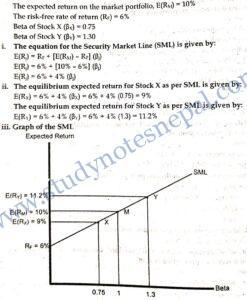###### 1. Explain why investors prefer to hold a diversified portfolio of securities. Also explain the contribution of Markowitz model on it.

A portfolio of investment means combining investment into more than one asset. For example, if we have Rs 100,000 for investing, we can invest all money in a single asset or more than one asset. If we invest in more than one asset, our investment is called a portfolio of assets. The basic idea behind forming a portfolio is to reduce investment risk. A portfolio can reduce risk sometimes even without sacrificing the return. In other words, a single asset can be very risky when it is held in isolation, but not much risky if it is held in combination with other assets in a portfolio. That means the return on portfolio may be more stable than the return on individual asset. It happens due to the diversification effect. By diversification we mean investments into two or more risk class assets.

If investors want to earn higher rate of return, they obviously must choose investments with higher risk. However, most investors do not hold just one asset; they hold a portfolio of assets. Hence, by diversification or investment in more than one asset, the riskiness of one asset can be influenced by the interaction of the pattern of its return with that of other assets it is held in combination. When an asset is held in isolation, the loss is more likely if the returns turn out to be very low. However, if two assets are held in combination, the returns on both must be low to suffer from a loss. Therefore through the diversification or investment in more than one risk class asset, where the assets’ returns do not move proportionally in the same direction at the same time, we can reduce the risk. For this reason, the investors prefer to hold a diversified portfolio of securities.

Diversification has the power of reducing risk. The power of diversification fundamentally depends on the correlation between assets in the portfolio. Concept of diversification is basically attributed to the contribution of Harry Markowitz. Markowitz suggests that an investor can achieve the benefit of risk reduction by combining assets with lower correlation in the portfolio. Thus, lower the correlation between assets, higher will be the benefit of risk reduction that a portfolio offers. Correlation is the statistical measure of relationship between two sets of data. It measures the degree to which two sets of data move together. The correlation between two sets of data may be positive or negative or zero. A positive correlation implies that two series move together into the same direction. Similarly, negative correlation indicates that two series move into opposite direction of each other. And, zero correlation implies that there is no relation between two sets of data. The covariance is an equivalent measure of relationship between two variables. The difference between covariance and correlation is that covariance shows the direction of relationship whereas correlation shows both direction as well as magnitude of relationship.

Markowitz Diversification is to combine the assets with lower correlation in a portfolio to obtain better benefit of risk reduction. According to this concept of diversification, if we are able to find securities with perfectly negative correlation, we will be able to eliminate the risk. It also suggests us that portfolio of securities with perfectly positive correlation does not reduce risk. In practice, there are hardly any securities with perfectly positive or perfectly negative correlations. Almost all securities have correlations between -1 and +1. Therefore, investing in a portfolio of securities with lower correlations better diversifies risk than investing in a portfolio of randomly selected securities. This is an important contribution of Markowitz model to the knowledge of investing.

It should be noted that portfolio average return is higher if we invest more of the portfolio fraction in assets yielding higher average return. However, this is not a case with portfolio risk because portfolio risk is not only the function of portfolio weight but also a function of covariance between the assets in the portfolio. If we form a portfolio of two assets, one with higher risk and other with lower risk, the portfolio risk first falls to a certain point, and then increases if we invest more in the asset with higher risk. This happens with all portfolios of assets having correlation between -1 and +1.

Further, Markowitz model shows that more portfolio risk reduction is possible if correlation between assets is closer to -1. And, if we allocate the appropriate portfolio weights into the assets, risk can even be reduced to zero when correlation is exactly -1. If two assets are perfectly negatively correlated, movement in returns from one asset is exactly offset by other, and hence the risk is eliminated. On the other hand, if two assets are perfectly positively correlated, movements in their returns do not offset each other at all, and hence the risk is the weighted average of the standard deviations of individual assets making up the portfolio. Thus the concept of covariance and correlation in Markowitz model is closely related to diversification.

###### 2.  Explain why most investors prefer to hold a diversified portfolio of securities as opposed to placing all of their wealth in a single asset. Also discuss why the concept of covariance is closely related to diversification.

A portfolio of investment means combining investment into more than one asset. For example, if we have Rs 100,000 for investing, we can invest all money in a single asset or more than one asset. If we invest in more than one asset, our investment is called a portfolio of assets. The basic idea behind forming a portfolio is to reduce investment risk. A portfolio can reduce risk sometimes even without sacrificing the return. In other words, a single asset can be very risky when it is held in isolation, but not much risky if it is held in combination with other assets in a portfolio. That means the return on portfolio may be more stable than the return on individual asset. It happens due to the diversification effect. By diversification we mean investments into two or more risk class assets.

If investors want to earn higher rate of return, they obviously must choose investments with higher risk. However, most investors do not hold just one asset; they hold a portfolio of assets. Hence, by diversification or investment in more than one asset, the riskiness of one asset can be influenced by the interaction of the pattern of its return with that of other assets it is held in combination. When an asset is held in isolation, the loss is more likely if the returns turn out to be very low. However, if two assets are held in combination, the returns on both must be low to suffer from a loss. Therefore through the diversification or investment in more than one risk class asset, where the assets’ returns do not move proportionally in the same direction at the same time, we can reduce the risk. For this reason, the investors prefer to hold a diversified portfolio of securities.

Diversification has the power of reducing risk. The power of diversification fundamentally depends on the correlation between assets in the portfolio. Concept of diversification is basically attributed to the contribution of Harry Markowitz. Markowitz suggests that an investor can achieve the benefit of risk reduction by combining assets with lower correlation in the portfolio. Thus, lower the correlation between assets, higher will be the benefit of risk reduction that a portfolio offers. Correlation is the statistical measure of relationship between two sets of data. It measures the degree to which two sets of data move together. The correlation between two sets of data may be positive or negative or zero. A positive correlation implies that two series move together into the same direction. Similarly, negative correlation indicates that two series move into opposite direction of each other. And, zero correlation implies that there is no relation between two sets of data. The covariance is an equivalent measure of relationship between two variables. The difference between covariance and correlation is that covariance shows the direction of relationship whereas correlation shows both direction as well as magnitude of relationship.

Markowitz Diversification is to combine the assets with lower correlation in a portfolio to obtain better benefit of risk reduction. According to this concept of diversification, if we are able to find securities with perfectly negative correlation, we will be able to eliminate the risk. It also suggests us that portfolio of securities with perfectly positive correlation does not reduce risk. In practice, there are hardly any securities with perfectly positive or perfectly negative correlations. Almost all securities have correlations between -1 and +1. Therefore, investing in a portfolio of securities with lower correlations better diversifies risk than investing in a portfolio of randomly selected securities. This is an important contribution of Markowitz model to the knowledge of investing.

It should be noted that portfolio average return is higher if we invest more of the portfolio fraction in assets yielding higher average return. However, this is not a case with portfolio risk because portfolio risk is not only the function of portfolio weight but also a function of covariance between the assets in the portfolio. If we form a portfolio of two assets, one with higher risk and other with lower risk, the portfolio risk first falls to a certain point, and then increases if we invest more in the asset with higher risk. This happens with all portfolios of assets having correlation between -1 and +1.

Further, Markowitz model shows that more portfolio risk reduction is possible if correlation between assets is closer to -1. And, if we allocate the appropriate portfolio weights into the assets, risk can even be reduced to zero when correlation is exactly -1. If two assets are perfectly negatively correlated, movement in returns from one asset is exactly offset by other, and hence the risk is eliminated. On the other hand, if two assets are perfectly positively correlated, movements in their returns do not offset each other at all, and hence the risk is the weighted average of the standard deviations of individual assets making up the portfolio. Thus the concept of covariance and correlation in Markowitz model is closely related to diversification.

3. On Jan 1, 2009 Ms. Pradhan invested Rs 100,000 in a portfolio of stocks and corporate bonds; Two-third (2/3) was placed into common stocks and remaining into corporate bonds.. On Dec. 31, 2009 Ms. Pradhan’s stocks and bonds holding were worth Rs 60,000 and Rs 34,000 respectively. During the year Rs 3,000 cash dividend were received on the stocks and Rs 2,720 in coupon payments, was received on the bonds. What was the return on Pradhan’s total portfolio during the year?

SOLUTION

Given: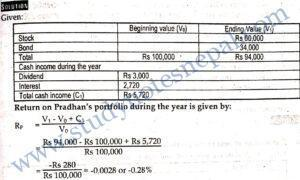4. On Jan 1, 2010 Ms. Kipu invested Rs 75,000 in a portfolio of stocks and corporate bonds. Rs 45,000 was placed into common stocks and the ce was in corporate bonds. On Dec. 31, 2010 Kipu’s stocks and bonds holding were worth Rs 40,000 and Rs 33,600 respectively. During the year Rs 2,000 cash dividend were received on the stocks and Rs 1,500 in coupon payments was received on the bonds. What was the return on Kipu’s total portfolio during the year?

SOLUTION

Given: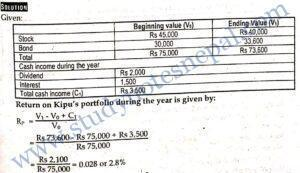5. Assume that the risk free rate is 6 percent, the expected return on the market over the next year is 10 percent, the beta of the common stock of Mercantile Computer is 1.75, and the beta of Beltronic Computer is 0.90. You are considering a portfolio of 100 shares Mercantile Computers and 150 shares of Beltronic Computer. The current price of a share of Mercantile Computer is Rs 380, and the price of a share of Beltronic Computer is Rs 200. Calculate:

1. The required rate of return for the common stock of Mercantile Computer.
2. The required rate of return for the common stock of Beltronic Computer.
3. The required rate of return for the portfolio.

SOLUTION

Given:

Risk free rate of return (RF) = 6%

Expected return on the market E(RM) = 10 %